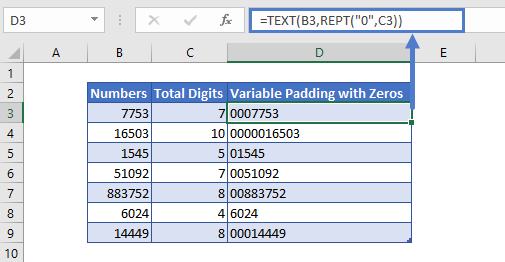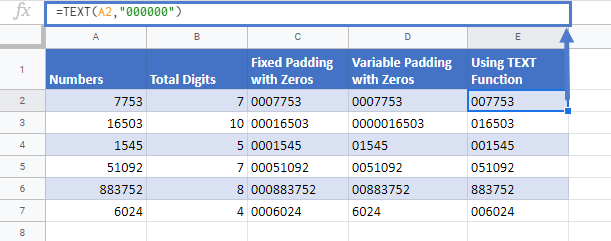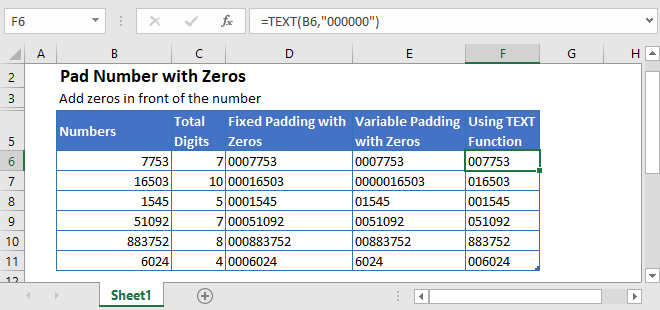## Numbers Stored as Text

There are several ways to add leading zeros to numbers in Excel. The method you want to use may depend on your end goal.

When considering adding leading zeros to numbers you need to decide if you want Excel to store your number as a number or as text. All of the examples, except this first example, will change your number to a “number stored as text”. This may or may not be what you want.

To adding leading zeros by changing number formatting, go to Number Formatting (CTRL + 1) > Custom and type 000000.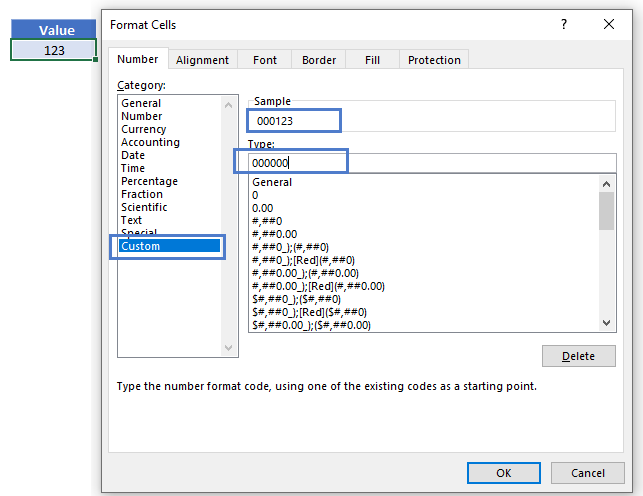This will ensure that all numbers are at least 6 digits long. If a number is less than 6 digits, leading zeros are added to the number: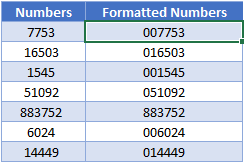Remember: This is the only method to add leading zeros to a number while keeping the number stored as a number in Excel.

The TEXT Function is used to convert numbers to text while applying specific number formatting. Here we can use the same number formatting as above “000000” to make all numbers at least 6 digits, just like the previous example.

However, these numbers are now stored as text (you can see column B the numbers are right-aligned, and in column C the numbers are left-aligned because they are stored as text):

``=TEXT(B3,"000000")``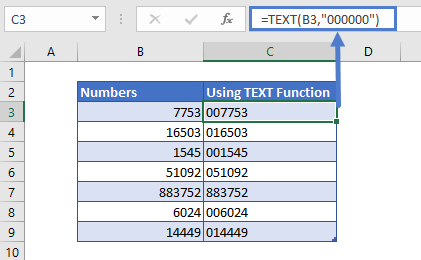## Add Fixed Number of Zeros

The above examples will add leading zeros only if the number is less than the specified number of digits. Instead, we can simply append a certain number of leading zeros using the ampersand operator (&) or the CONCATENATE Function.

### Using Ampersand Operator

The ampersand operator joins together strings of text. Here we used & to add three leading zeros to our numbers:

``="000"&B3``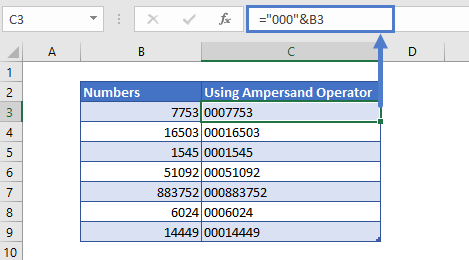### Using the CONCATENATE Function

The CONCATENATE Function can also join together strings of text:

``=CONCATENATE("000",B3)``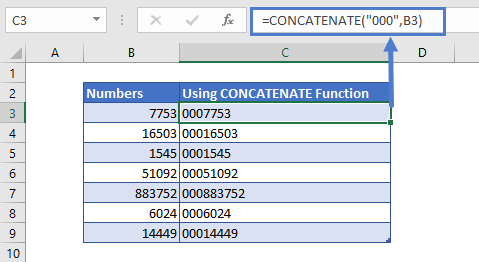### Easy Excel Automation## REPT Function – To Specify Number of Zeros

The REPT Function repeats a character (or character) a number of times. We can use the REPT Function to generate a number of leading zeros or with the TEXT Function to specify the total number of digits a number should have.

This is extremely useful as it saves you from counting out the number of zeros you’d like to add, and the potential for an error.

Here is an example of the REPT Function inside the TEXT Function:

``=TEXT(B3,REPT("0",C3))``